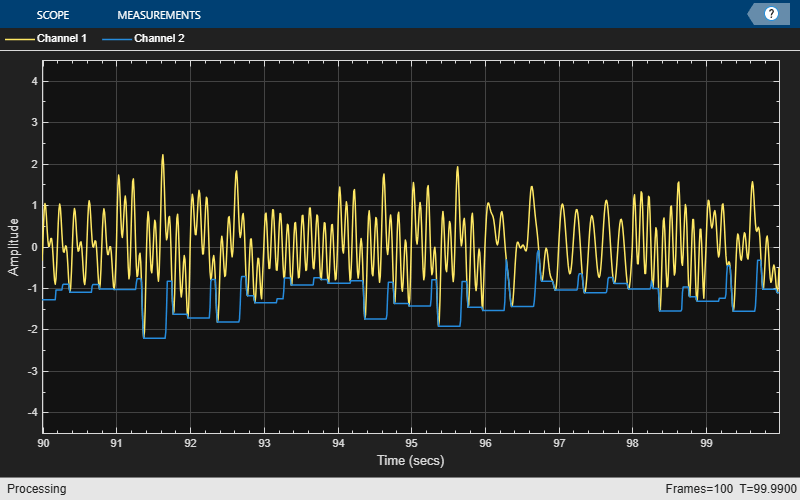# dsp.MovingMinimum

Moving minimum

## Description

The `dsp.MovingMinimum` System object™ determines the moving minimum of the input signal along each channel, independently over time. The object uses the sliding window method to determine the moving minimum. In this method, a window of specified length is moved over each channel, sample by sample, and the object determines the minimum of the data in the window. For more details, see Algorithms.

To determine the moving minimum of the input:

1. Create the `dsp.MovingMinimum` object and set its properties.

2. Call the object with arguments, as if it were a function.

## Creation

### Syntax

``movMin = dsp.MovingMinimum``
``movMin = dsp.MovingMinimum(Len)``
``movMin = dsp.MovingMinimum(Name,Value)``

### Description

````movMin = dsp.MovingMinimum` returns a moving minimum object, `movMin`, using the default properties.```

example

````movMin = dsp.MovingMinimum(Len)` sets the `WindowLength` property to `Len`.```
````movMin = dsp.MovingMinimum(Name,Value)` specifies additional properties using `Name,Value` pairs. Unspecified properties have default values.Example: ```movMin = dsp.MovingMinimum('SpecifyWindowLength',1,'WindowLength',10);``````

## Properties

expand all

Unless otherwise indicated, properties are nontunable, which means you cannot change their values after calling the object. Objects lock when you call them, and the `release` function unlocks them.

If a property is tunable, you can change its value at any time.

Flag to specify a window length, specified as a scalar boolean.

• `true` — The length of the sliding window is equal to the value you specify in the `WindowLength` property.

• `false` — The length of the sliding window is infinite. In this mode, the object determines the minimum of the current sample and all the past samples.

Length of the sliding window in samples, specified as a positive scalar integer.

#### Dependencies

This property applies when you set `SpecifyWindowLength` to `true`.

Data Types: `single` | `double` | `int8` | `int16` | `int32` | `int64` | `uint8` | `uint16` | `uint32` | `uint64`

## Usage

### Syntax

``y = movMin(x)``

### Description

example

````y = movMin(x)` determines the moving minimum of the input signal, `x`, using the sliding window method.```

### Input Arguments

expand all

Data input, specified as a vector or a matrix. If `x` is a matrix, each column is treated as an independent channel. The moving minimum is determined along each channel. The object also accepts variable-size inputs. Once the object is locked, you can change the size of each input channel, but you cannot change the number of channels.

Data Types: `single` | `double` | `int8` | `int16` | `int32` | `int64` | `uint8` | `uint16` | `uint32` | `uint64`

### Output Arguments

expand all

Moving minimum output, returned as a vector or a matrix.

Data Types: `single` | `double` | `int8` | `int16` | `int32` | `int64` | `uint8` | `uint16` | `uint32` | `uint64`

## Object Functions

To use an object function, specify the System object as the first input argument. For example, to release system resources of a System object named `obj`, use this syntax:

`release(obj)`

expand all

 `step` Run System object algorithm `release` Release resources and allow changes to System object property values and input characteristics `reset` Reset internal states of System object

## Examples

collapse all

Compute the moving minimum of a sum of three sine waves with varying amplitude. Use a sliding window of length 30.

Initialization

Set up an input signal that is a sum of three sine waves with frequencies at 2 Hz, 5 Hz, and 10 Hz. The sampling frequency is 100 Hz. Create a `dsp.MovingMinimum` object with a window length of 30. Create a time scope for viewing the output.

```sin = dsp.SineWave('SampleRate',100,... 'Frequency',[2 5 10],... 'SamplesPerFrame',100); movMin = dsp.MovingMinimum(30); scope = timescope('SampleRate',100,... 'TimeSpanSource','property','TimeSpan',10,... 'TimeSpanOverrunAction','Scroll',... 'ShowGrid',true,'YLimits',[-4.5 4.5]); ```

Compute the Moving Minimum

Each sine wave component of the input signal has a different amplitude that varies with the iteration. Use the `movMin` object to determine the minimum value of the current sample and the past 29 samples of the input signal.

```for index = 1:100 sin.Amplitude = rand(1,3); x = sum(sin(),2); xmin = movMin(x); scope([x,xmin]) end scope.AxesScaling = 'onceatstop'; ```## Algorithms

expand all

 Bodenham, Dean. “Adaptive Filtering and Change Detection for Streaming Data.” PH.D. Thesis. Imperial College, London, 2012.

## SupportGet trial now